## In order to study the long-term effects of weightlessness, astronauts in space must be weighed (or at least “massed”). One way in which this

Question

In order to study the long-term effects of weightlessness, astronauts in space must be weighed (or at least “massed”). One way in which this is done is to seat them in a chair of known mass attached to a spring of known force constant and measure the period of the oscillations of this system. The 36.4-kg chair alone oscillates with a period of 1.40 s, and the period with the astronaut sitting in the chair is 2.22 s.

A)Find the force constant of the spring?
B)Find the mass of the astronaut ?

in progress 0
2 months 2021-07-28T08:11:41+00:00 1 Answers 1 views 0

Explanation:

This question is based on oscillation in spring – mass system

time period is given by the relation T = 2π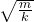m is mass attached , k is spring constant of spring .

In the first case

mass m = 36.4 kg , T = 1.4

Putting these values in the equation above

1.4 = 2π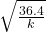1.4² = 4π² x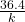k = 732.42 N / m

In the second case

mass m = (36.4+m ) kg , T = 2.22s  , m is mass of astronaut

Putting these values in the equation above

2.22  = 2π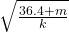2.22² = 4π² x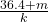Putting the value of k

2.22² = 4π² x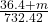36.4 + m = 91.53

m = 55.12.kg